Home/Blog/Interview Prep/7 of the most important LeetCode patterns for coding interviews

# 7 of the most important LeetCode patterns for coding interviews

Nov 01, 2022 - 14 min readHunter JohnsonMastering coding techniques, usable in solving a large number of computational problems, is critical to thriving in a current job as well as securing a new position in your favorite company.

Some developers spend time and effort to find a new solution to each computational problem from scratch. In contrast, you can save time by quickly finding an efficient and robust solution using existing techniques.

Preparing intelligently by focusing on problem-solving skills and underlying design patterns will make all the difference in the competitive coding interview, whether it’s with a FAANG company or not.

LeetCode has proven itself as a great resource and repository for practicing a large number of coding interview questions. This kind of practice is necessary for those preparing for interviews. But without considering the patterns associated with each question and answer, such an effort is futile given the sheer vastness of the computing subject.

In this article, we will cover some of the most popular coding interview patterns to help you organize your LeetCode coding interview preparation. Our structured and organized approach will lead to a greater understanding of the overarching concepts and patterns you’ll be practicing.

Let’s get started!

We’ll cover:

## What is LeetCode?

LeetCode is a website where learners can practice solving computational problems that are common in coding interviews. LeetCode has over 2,000 questions for you to practice, covering various concepts with a deep roster of supported programming languages. Each coding problem has a difficulty scale of Easy, Medium, or Hard. The problems focus on data structures and algorithms.

Here are some example problems you can find:

• Two sum problem
• Sorting arrays
• Largest common subsequences
• Valid parentheses
• Search 2D matrix
• Valid anagrams
• Binary Search Tree (BST)
• Level order traversal
• Longest substring without repeating characters
• Valid palindromes
• Path sum

## Recognizing patterns in techniques and structure

Instead of crushing hundreds of loosely related coding interview problems daily, try completing problem sets that follow the same data structure and/or algorithmic technique. Finding success with LeetCode is not about how many problems you can complete before the interview but rather about learning the structures and techniques. Once you’re familiar with patterns, then you can use that knowledge to solve a multitude of related problems instead of just the few you encounter on LeetCode.

In other words, coding patterns are a path to connecting an unknown problem to a known problem. Let’s look at some of these major patterns and how to use them in practice.

## 1. Two pointers technique

When solving problems with sorted arrays that require fulfilling certain constraints with a specific set of elements, the two pointers technique becomes quite handy. The set of elements could be a pair, a triplet, or even a subarray.

Take a look at this example problem:

Given an array of sorted numbers and a target sum, find a pair in the array whose sum is equal to the given target.

To solve this problem, a naive approach is to use two nested loops and check all the possible pairs to find the one whose sum is equal to the target value. Each of the nested loops will be running to the full length of the array. Thus, the time complexity of this algorithm is $O(N^2)$, where N is the number of elements in the input array. You could do much better than the above approach using the two pointers technique. Let’s assume that the input array is sorted starting with the first pointer at the beginning and the second pointer at the end.

At every step, you will see if the numbers pointed by the two pointers add up to the target sum. If so, then you return the two values pointed out by them. Otherwise, you will do one of two things:

• If the sum of the two numbers pointed by the two pointers is greater than the target sum, this means that you need a pair with a smaller sum. So, to try more pairs, you can move the second pointer toward the left side of the array.
• If the sum of the two numbers pointed by the two pointers is smaller than the target sum, this means that you need a pair with a larger sum.

So, to try more pairs, you can move the first pointer toward the right side of the array.

The two pointers technique has decreased the time complexity of the approach to $O(N)$. Thus, it is a more efficient approach to finding a pair with the target sum in an array.

## 2. Sliding windows

In many problems dealing with an array or linked list, you are asked to find or calculate something among all the subarrays of a given size. A window is a sublist formed over a part of an iterable data structure. It can be used to slide over the data in chunks corresponding to the window size. For example, take a look at this problem:

Given an array, find the average of each subarray of ‘K’ contiguous elements in it.

Let’s understand this problem with an example:

Array: [1, 3, 2, 6, -1, 4, 1, 8, 2], K=5

Here, you are asked to find the average of all subarrays of 5 contiguous elements in the given array. Let’s solve this: For the first 5 numbers (subarray from index 0-4), the average is: (1+3+2+6-1)/5 => 2.2

The average of the next 5 numbers (subarray from index 1-5) is: (3+2+6-1+4)/5 => 2.8

For the next 5 numbers (subarray from index 2-6), the average is: (2+6-1+4+1)/5 => 2.4

And so on.

Here is the final output containing the averages of all subarrays of size 5:

Output: [2.2, 2.8, 2.4, 3.6, 2.8]

Let’s discuss two possible solutions for the sliding window technique.

### Brute force

You can use two nested loops where the outer loop is going through each window, and the inner loop is finding the maximum from that window. The worst-case time complexity of such an approach is $O(N\times K)$, given $N$ is the number of elements in the array, and $K$ is the size of the window.

### Balanced binary search tree

A better approach is to use a binary search tree. You start by inserting elements of the first window in a self-balancing binary search tree, such as an AVL tree. Now, every time the window slides, we create a new window by removing a node and inserting a new node in the tree. As it is a balanced binary search tree, the actions of insertion, deletion, and finding the maximum each requires only $O(\log(K))$ time, where $K$ is the number of elements in a window. Therefore, finding the maximums from trees has the overall worst-case complexity of $O(N \log(K))$, where $N$ is the number of elements in the array.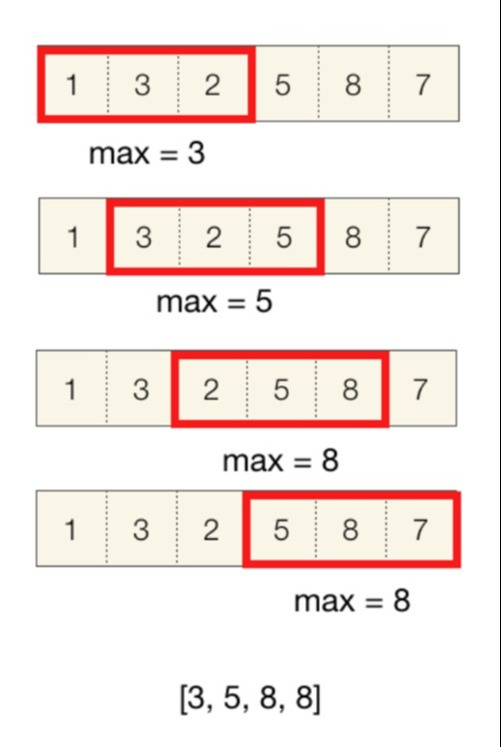Visual example of a different sliding windows problem

## 3. 0/1 Knapsack Pattern

The 0/1 Knapsack pattern is based on the well-known problem with the same name, which is solved using dynamic programming. When given the weights and profits of ‘N’ items, you are asked to put these items in a “knapsack” with a capacity ‘C.’ The goal is to get the optimum profit out of the items in the knapsack.

Let’s take a look at a simple example. Let’s assume a store owner wants to carry fruits in the knapsack in a way that returns the maximum profit possible.

Weights and profits of the fruits:

Items: { Apple, Orange, Banana, Melon }

Weights: { 2, 3, 1, 4 }

Profits: { 4, 5, 3, 7 }

Knapsack capacity: 5

Try to place various combinations in the knapsack so that the total weight is not more than 5. Some of these combinations would include:

• Apple + Orange (total weight 5) => 9 profit
• Apple + Banana (total weight 3) => 7 profit
• Orange + Banana (total weight 4) => 8 profit
• Banana + Melon (total weight 5) => 10 profit

As you can see, Banana and Melon yields the highest profit without exceeding the maximum weight.

So, if you’re given two integer arrays to represent the profits and weights of N items, you then need to find the subset of these items that will produce a maximum profit that is not more than the given C value. Each item can only be selected once, meaning it is either selected for the knapsack or skipped.

It helps to start with a brute-force recursive solution to gauge the overlapping sub-issues. After the recursive solution, try the advanced techniques of Memoization and Bottom-Up Dynamic Programming to complete the full pattern.

Here is an example algorithm of a brute-force approach revealing all combinations of a set, with the example items of A, B, C, and D:

for each item 'i'  create a new set which INCLUDES item 'i' if the total weight does not exceed the capacity, and     recursively process the remaining capacity and items  create a new set WITHOUT item 'i', and recursively process the remaining itemsreturn the set from the above two sets with higher profit

The time complexity of this approach is $O(2^n)$.

## 4. Fast and slow pointers pattern

The fast and slow approach is commonly referred to as the Hare and Tortoise algorithm. It is a pointer algorithm that utilizes two pointers that move through the array, sequence, or linked list at varying speeds.

This approach is most commonly used to deal with cyclic linked lists and to determine if the linked list contains a cycle or not. It’s also called Floyd’s cycle detection. Let’s walk through a simple example. You create two pointers, namely, a slow pointer and a fast pointer. In each set, the slow pointer moves one step, and the fast pointer moves two steps.

This gives us two conclusions:

If the linked list doesn’t have a cycle, the fast pointer will reach the end of the linked list before the slow pointer to prove that there is no cycle in the linked list.

The slow pointer will not be able to catch up to the fast pointer if there is indeed no cycle in the linked list. If the linked list has a cycle, the fast pointer enters the cycle first, followed by the slow pointer. Both pointers will keep moving in the cycle infinitely. If at any stage, both of these pointers meet, you can confidently conclude that the linked list has a cycle in it. It is up to you to determine if it’s possible for the two pointers to meet.

When the fast pointer is approaching the slow pointer from behind, we have two possibilities:

1. The fast pointer is one step behind the slow pointer.
2. The fast pointer is two steps behind the slow pointer.

Other distances between the fast and slow pointers will become one of these two possibilities.

Let’s analyze these scenarios, considering the fast pointer always moves first:

If the fast pointer is one step behind the slow pointer: The fast pointer moves two steps, and the slow pointer moves one step, and they both meet.

If the fast pointer is two steps behind the slow pointer: The fast pointer moves two steps, and the slow pointer moves one step. After the moves, the fast pointer will be one step behind the slow pointer, reducing this to the first scenario. This means that the two pointers will meet in the next series.

This concludes that the two pointers will definitely meet if the linked list has a cycle.

The time complexity of the technique will be $O(n)$. ‘n’ will be the total number of nodes.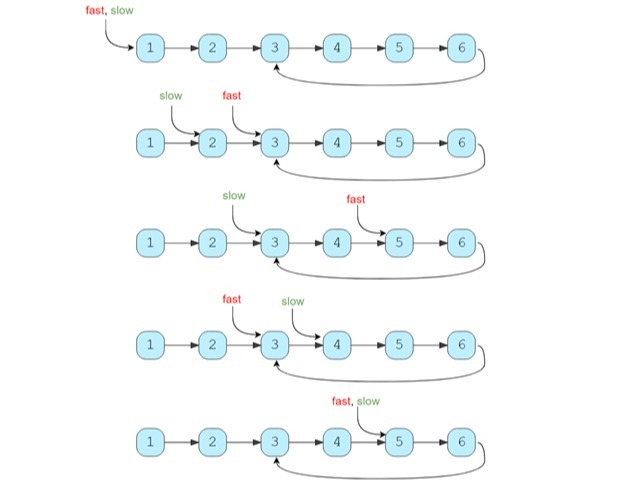An illustration of the fast and slow pointers pattern using a linked list with a cycle

## 5. Merge Intervals

This pattern describes a technique to deal with overlapping intervals. In many problems you’ll face involving intervals, you’ll either need to find overlapping intervals or merge intervals if they overlap. Given two intervals (‘a’ and ‘b’), there will be six different ways the two intervals can relate to each other.

This illustration below shows all six possibilities.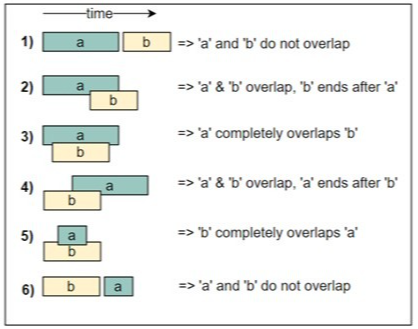Becoming familiar with the above six cases will assist you in solving all interval-related problems.

Here is a quick example of a related question:

Given a list of intervals, merge all the overlapping intervals to produce a list with only mutually exclusive intervals.

Intervals: [[1,4], [2,5], [7,9]]Output: [[1,5], [7,9]]Explanation: Since the first two intervals [1,4] and [2,5] overlap, we merged them into one [1,5].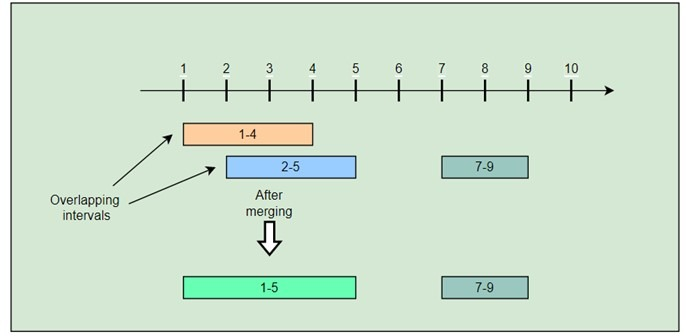## 6. In-place reversal of a linked list

In many coding interview problems, the participant is asked to reverse the links between a set of nodes from a linked list. The challenge often arises from doing this “in-place,” meaning with the existing node objects and no extra memory storage.

This pattern details an effective way to solve these pesky problems. Let’s look at an example in C++:

Given a singly linked list, reverse it in place, that is, without using additional memory

Solution:

You traverse the linked list while keeping track of three nodes: the current node, the previous node, and the next node. Keeping track of these nodes will allow you to reverse the linked list in place during the traversal. To elaborate, you point the current node to the previous one. Subsequently, the next node becomes the current node, and the process continues.

This method will use $O(1)$ space and will allow you to reverse the linked list in a single traversal. Thus, the worst-case time complexity of this method is $O(N)$ where ‘N’ is the number of nodes in the linked list.

## 7. Tree Breadth-First Search (BFS)

The Breadth-First Search (BFS) algorithm is used for traversing and searching tree or graph data structures. All the nodes are explored at the present depth before moving to the nodes at the next depth level. The BFS algorithm is usually implemented using a queue.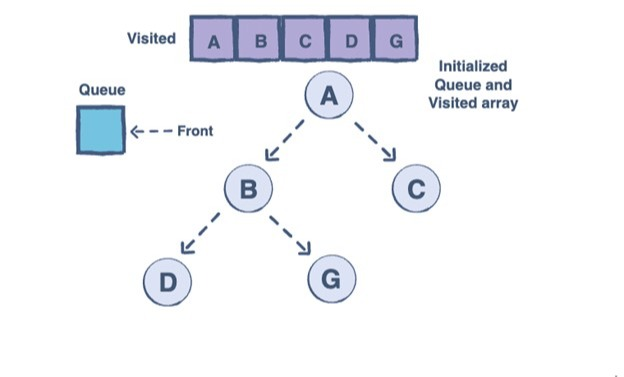Any problem involving the traversal of a tree in a level-by-level order can be efficiently solved using this approach. You will use a queue to keep track of and efficiently traverse all the nodes of a level before jumping onto the next level. This also means that the space complexity of the algorithm will be O(W), where ‘W’ is the maximum number of nodes on any level.

Here are the steps of the algorithm:

1. Start by pushing the root node to the queue.
2. Keep iterating until the queue is empty.
3. In each iteration, first count the elements in the queue (let’s call it levelSize). We will have this many nodes at the current level.
4. Next, remove levelSize nodes from the queue and push their value into an array to represent the current level.
5. After removing each node from the queue, insert both of its children into the queue.
6. If the queue is not empty, repeat step 3 for the next level.

“Giving someone a fish will feed them for a day but teaching them to fish will feed them for life” is a popular saying that rings true to the coding interview. Catching a few fish does not matter if you can’t replicate that success in a strange pond, because skilled professionals can catch a fish no matter the conditions.

Companies are looking to hire skilled developers who can solve even the most unfamiliar of problems. Dedicated practice of these concepts is key because, as we all know, the greatest developers (and anglers!) don’t become great overnight! Solving a few problems involving reversing a linked list or merging intervals and simply moving on to the next will teach you how to solve those specific problems. By understanding the relevant underlying technique, you’ll gain the know-how to solve virtually any problem involving reversing a linked list or merging intervals.

a few other valuable interview patterns that we didn’t discuss in-depth are:

Learning the coding design patterns and algorithms underlying specific questions will tremendously help you use LeetCode and other interview prep sources to their maximum potential!

To continue preparing efficiently with these popular interview prep patterns, check out our new Grokking Coding Interview Patterns in Python course! It covers the seven approaches discussed in this article, and many others, in detail.

Happy learning!

### Continue learning about patterns and interview prepWRITTEN BYHunter Johnson

Join a community of 1.7 million readers. Enjoy a FREE, weekly newsletter rounding up Educative's most popular learning resources, coding tips, and career advice.

Learn in-demand tech skills in half the time